In this blog we will try to understand K Nearest Neighbors (KNN) algorithm which is used to classify things. Let’s start with two bunches of dots shown below.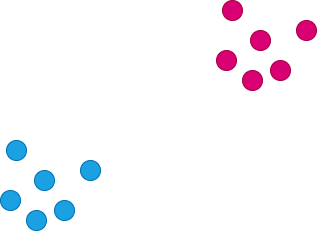Bunch of sots

One set is red and other set is blue. Now we have got a dot at an location X as shown below.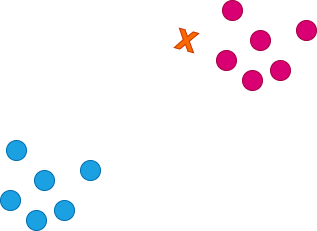what would X be?

What color the dot at X should be? For us humans its simple, we know it should be a red dot because it’s near the red cluster, but now we have to tell computer how to find it out.

To give the computer this intelligence, we first tell it to compute the distance from X to all the dots.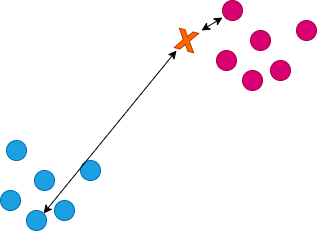Compute distance of X to dots

Naturally the distance from X to red dots will be the least and the blue ones will be farthest.

Now we order the dots from the least to most farthest distance as shown below.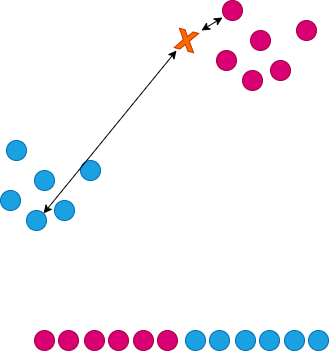Order dots by distance

Now we choose our K which in this case we choose 8, it should be a educated guess, the K is nothing but a number chosen that will pick the K least distance (or nearest neighbors) dots from X.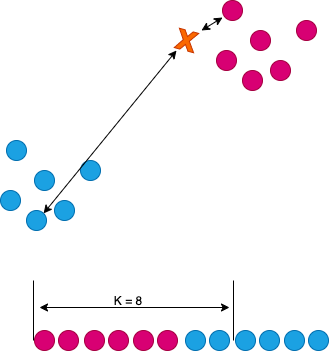Choosing K

Now in K nearest dots we count how many of them are red and how many of them are blue. For us 6 of them are red and and 2 of them are blue as shown below.Vote the winner

So there are more votes for red, so the dot at X should be red.

One may get the diagrams for this blog here knn.drawio